Next: Optimization codes and modeling Up: Discretization and optimization techniques Previous: Discretization and optimization techniques

## Discretization approach

The discussion of discretization schemes is restricted to the standard situation where the domain is the unit square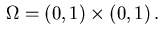The purpose of this section is to develop discretization techniques by which the distributed control problem (2.1)-(2.6) is transformed into a nonlinear programming problem (NLP-problem) of the form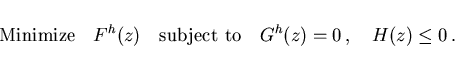(3.1)

The functions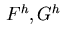andare sufficiently smooth and are of appropriate dimension. The upper subscript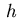denotes the dependence on the stepsize. The optimization variable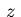will comprise both the discretized state and control variables.

The form (3.1) will be achieved by solving the elliptic equation (2.2) with the standard five-point-star discretization scheme. Choose a number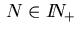and the stepsize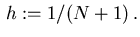Consider the mesh points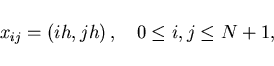and define the following sets of indices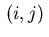residing either in the domain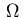or on the boundary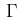, resp. on the subsets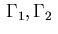of the boundary: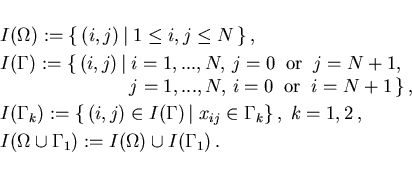(3.2)

Obviously, we have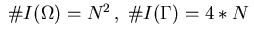; define further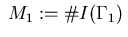.

Now we shall present discretization schemes for the distributed control problem that are similar to those for boundary controls considered in Part 1 . The optimization variablein (3.1) is taken as the vector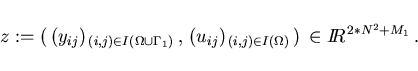The remaining state variables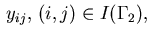are are determined by the Dirichlet condition (2.4) as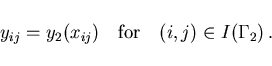(3.3)

The derivative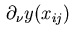in the direction of the outward normal is approximated by the expression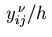where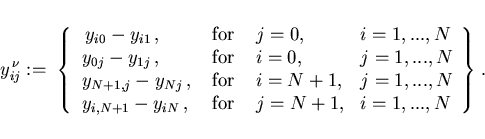(3.4)

Then the discrete form of the Neumann boundary condition (2.3) leads to the equality constraints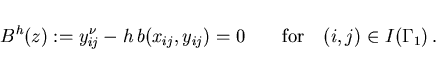(3.5)

The application of the five-point-star to the elliptic equation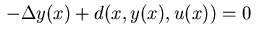in (2.2) yields the following equality constraints for all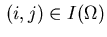: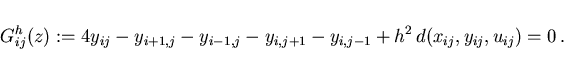(3.6)

Note that the Dirichlet condition (3.3) is used in this equation to substitute the variables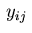for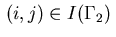. The control and state inequality constraints (2.5) and (2.6) yield the inequality constraints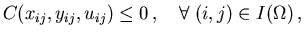(3.7)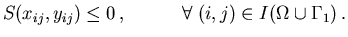(3.8)

Observe that these inequality constraints do not depend on the meshsize. The discretized form of the cost function (2.1) is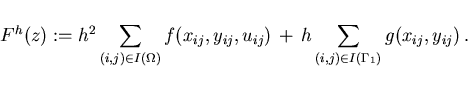(3.9)

In summary, the relations (3.5)-(3.9) define a NLP-problem of the form (3.1). The corresponding Lagrangian function is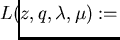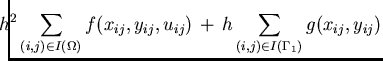(3.10)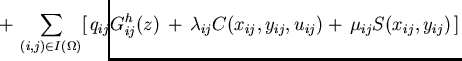where the Lagrange multipliers,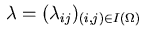and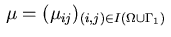are associated with the equality constraints (3.5) and (3.6), resp. the inequality constraints (3.7) and (3.8). In addition, the multipliers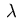and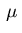satisfy the complementarity conditions corresponding to (2.14):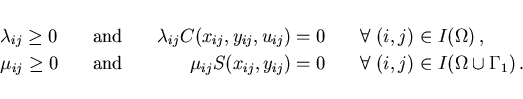In the next step we discuss the necessary conditions of optimality: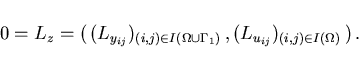For state variableswith indices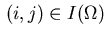we obtain the relations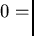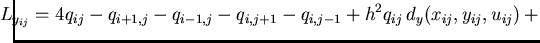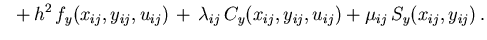(3.11)

In these equations, the up to now undefined multipliers are set to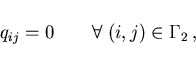(3.12)

which is in accordance with the Dirichlet condition (2.12). We deduce from equations (3.11) that the Lagrange multipliers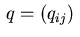satisfy the five-point-star difference equations for the adjoint equation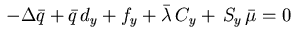in (2.10) if we use the following approximations for the multiplier function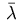and the regular Borel measure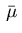,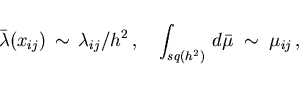(3.13)

where in the second relation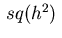denotes a square centered at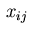with area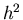. Recall the decomposition (2.15) of the measure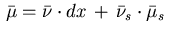. If the singular part of the measure vanishes, i.e.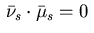holds, then (3.13) yields the following approximation for the density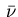,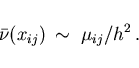(3.14)

In case that the measure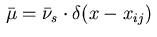is a delta distribution, we obtain from (3.13) the approximation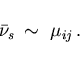(3.15)

For indices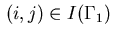on the boundary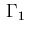, e.g., for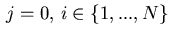, we obtain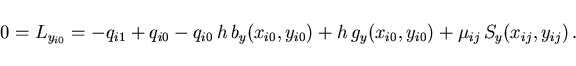These relations represent the discrete version of the Neumann boundary condition (2.11) if we approximate the regular Borel measureon the boundary by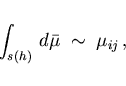(3.16)

where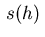denotes a line segment of lengthoncentered at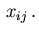If the singular part in the decomposition (2.15) vanishes resp. if the measureis a delta distribution, we obtain the following approximations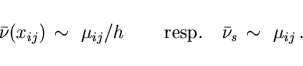(3.17)

Finally, necessary conditions with respect to the control variablesforare determined by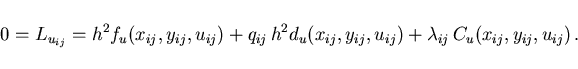From this equation we recover the discrete version of the control law (2.13), if we use the same identification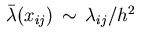as in (3.13).Next: Optimization codes and modeling Up: Discretization and optimization techniques Previous: Discretization and optimization techniques
Hans D. Mittelmann
2000-10-06